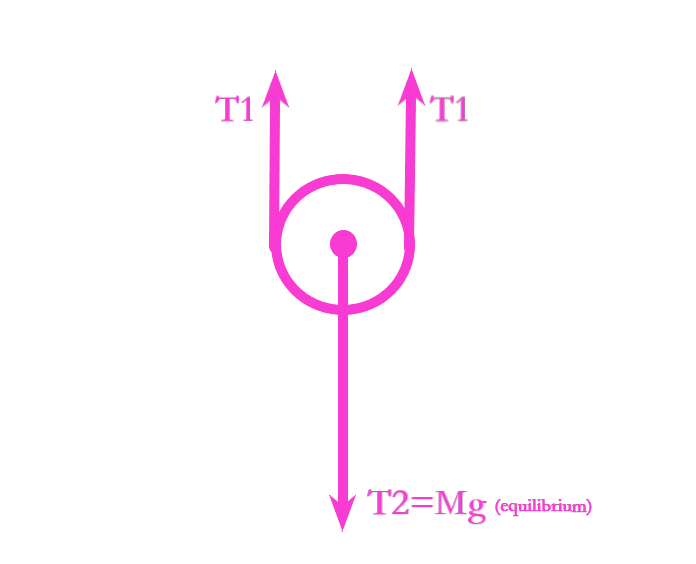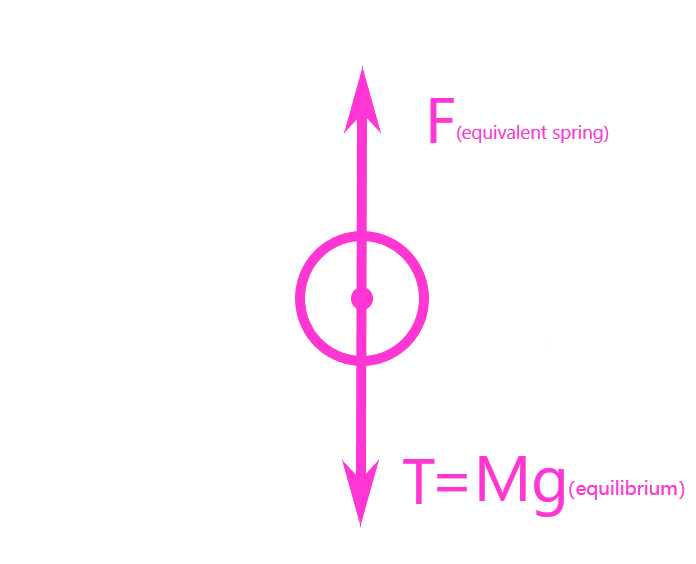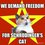# Simpler solution of Mechanics Warmups: Level 4 Challenges, problem 3Since my friends and I are taking non-calculus based Physics classes, we were shocked by the solutions given for their complexity. We found out a much simpler way to solve the problem--even people who have just learnt AP Physics 1 can understand.

First, assume that at the initial conditions (before oscillations start), both of the springs are at their initial lengths. At the instance when the pulley-spring-rope-mass system reaches equilibrium, Free-body Diagram of the movable pulley is shown below, (image 1) where tension in the rope with springs is T1 while tension in the rope to hang the mass is T2. The ropes are massless, so tension should have the same magnitude in a rope.

At that point, the movable pulley is in state of equilibrium so the net force acting on it is zero, which means taking upward to be positive)
Fnet=2T1-Mg= 0,
T1=Mg/2.

Assume that the springs are uniform. By Hooke’s Law, displacements of the springs have magnitude of
ΔX1=F1/K1=T/K1=Mg/2K1 and
ΔX2= F2/K2=T/K2=Mg/2K2, respectively.
The lengths of ropes are unchanged, so the pulley’s displacement from initial position has a magnitude of
ΔXpulley=total increased length of springs(and ropes, which equals 0)=(ΔX1+ΔX2)/2=( Mg/2K1+ Mg/2K2)/2.

Then, consider the springs to be one equivalent spring, whose spring constant can’t be obtained directly from method of connecting springs in series or in parallel (the two springs’ amplitudes are DIFFERENT in the oscillation). Forces acting on the movable pulley can be now considered as: (image 2)

The net force again is zero, so
F equivalent spring=T2=Mg.
Apply Hooke’s Law for a second time,
K equivalent spring=F/ΔX
=F equivalent spring/ΔXpulley=4K1K2/(K1+K2).
Using quantities above, we can get period of the system’s oscillation is
T=2π√(M/K)= 2π√(M/K equivalent spring)= 2π√(M(K1+K2)/4K1K2)
≈0.7695s.Note by Veronica Luo
3 months ago

This discussion board is a place to discuss our Daily Challenges and the math and science related to those challenges. Explanations are more than just a solution — they should explain the steps and thinking strategies that you used to obtain the solution. Comments should further the discussion of math and science.

When posting on Brilliant:

• Use the emojis to react to an explanation, whether you're congratulating a job well done , or just really confused .
• Ask specific questions about the challenge or the steps in somebody's explanation. Well-posed questions can add a lot to the discussion, but posting "I don't understand!" doesn't help anyone.
• Try to contribute something new to the discussion, whether it is an extension, generalization or other idea related to the challenge.

MarkdownAppears as
*italics* or _italics_ italics
**bold** or __bold__ bold
- bulleted- list
• bulleted
• list
1. numbered2. list
1. numbered
2. list
Note: you must add a full line of space before and after lists for them to show up correctly
paragraph 1paragraph 2

paragraph 1

paragraph 2

[example link](https://brilliant.org)example link
> This is a quote
This is a quote
    # I indented these lines
# 4 spaces, and now they show
# up as a code block.

print "hello world"
# I indented these lines
# 4 spaces, and now they show
# up as a code block.

print "hello world"
MathAppears as
Remember to wrap math in $$ ... $$ or $ ... $ to ensure proper formatting.
2 \times 3 $2 \times 3$
2^{34} $2^{34}$
a_{i-1} $a_{i-1}$
\frac{2}{3} $\frac{2}{3}$
\sqrt{2} $\sqrt{2}$
\sum_{i=1}^3 $\sum_{i=1}^3$
\sin \theta $\sin \theta$
\boxed{123} $\boxed{123}$

Sort by:

- 3 months ago

Sure, I'll try to fix it. Thanks! :)

- 3 months ago